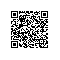# 人人都应该用的 Python 开源库

class Point3D(object):


class Point3D(object):
def __init__(self, x, y, z):



class Point3D(object):
def __init__(self, x, y, z):
self.x



class Point3D(object):
def __init__(self, x, y, z):
self.x = x



... 为 x ？嗯，很明显 ...

class Point3D(object):
def __init__(self, x, y, z):
self.x = x
self.y = y
self.z = z



... 现在我必须为每个属性做一次，所以实际上这个 尺度 真的把握的很糟糕吗？我一定要每个属性都这样输入 3 次吗 ？！

class Point3D(object):
def __init__(self, x, y, z):
self.x = x
self.y = y
self.z = z
def __repr__(self):



class Point3D(object):
def __init__(self, x, y, z):
self.x = x
self.y = y
self.z = z
def __repr__(self):
return (self.__class__.__name__ +
("(x={}, y={}, z={})".format(self.x, self.y, self.z)))



class Point3D(object):
def __init__(self, x, y, z):
self.x = x
self.y = y
self.z = z
def __repr__(self):
return (self.__class__.__name__ +
("(x={}, y={}, z={})".format(self.x, self.y, self.z)))
def __eq__(self, other):
if not isinstance(other, self.__class__):
return NotImplemented
return (self.x, self.y, self.z) == (other.x, other.y, other.z)



7 次?!

class Point3D(object):
def __init__(self, x, y, z):
self.x = x
self.y = y
self.z = z
def __repr__(self):
return (self.__class__.__name__ +
("(x={}, y={}, z={})".format(self.x, self.y, self.z)))
def __eq__(self, other):
if not isinstance(other, self.__class__):
return NotImplemented
return (self.x, self.y, self.z) == (other.x, other.y, other.z)
def __lt__(self, other):
if not isinstance(other, self.__class__):
return NotImplemented
return (self.x, self.y, self.z) < (other.x, other.y, other.z)



9 次?!

from functools import total_ordering
@total_ordering
class Point3D(object):
def __init__(self, x, y, z):
self.x = x
self.y = y
self.z = z
def __repr__(self):
return (self.__class__.__name__ +
("(x={}, y={}, z={})".format(self.x, self.y, self.z)))
def __eq__(self, other):
if not isinstance(other, self.__class__):
return NotImplemented
return (self.x, self.y, self.z) == (other.x, other.y, other.z)
def __lt__(self, other):
if not isinstance(other, self.__class__):
return NotImplemented
return (self.x, self.y, self.z) < (other.x, other.y, other.z)



from unittest import TestCase
class Point3DTests(TestCase):



## namedtuple 救援（不是真正意义上的）。

• 他们通过编号指标进行访问无论您是否希望这样做。除此之外，这意味着你不能有私有属性，因为他们通过明显的公共接口 __getitem__ 暴露出来。
• 它比较相等的值相同的原始 tuple ，所以很容易陷入离奇的类型混乱，特别是如果你想用它来使用 tuple 和 list 进行迁移。
• 这是一个元组，所以它 总是 一成不变的。 至于最后一点，你可以像它这样使用：
Point3D = namedtuple('Point3D', ['x', 'y', 'z'])



class Point3D(namedtuple('_Point3DBase', 'x y z'.split()])):
pass



## 键入 attr

import attr
@attr.s


import attr
@attr.s
class Point3D(object):



import attr
@attr.s
class Point3D(object):
x = attr.ib()



import attr
@attr.s
class Point3D(object):
x = attr.ib()
y = attr.ib()
z = attr.ib()



>>> Point3D(1, 2, 3)
Point3D(x=1, y=2, z=3)



>>> Point3D(1, 2, 3) == Point3D(1, 2, 3)
True
>>> Point3D(3, 2, 1) == Point3D(1, 2, 3)
False
>>> Point3D(3, 2, 3) > Point3D(1, 2, 3)
True



>>> attr.asdict(Point3D(1, 2, 3))
{'y': 2, 'x': 1, 'z': 3}



>>> import pprint
>>> pprint.pprint(attr.fields(Point3D))
(Attribute(name='x', default=NOTHING, validator=None, repr=True, cmp=True, hash=True, init=True, convert=None),
Attribute(name='y', default=NOTHING, validator=None, repr=True, cmp=True, hash=True, init=True, convert=None),
Attribute(name='z', default=NOTHING, validator=None, repr=True, cmp=True, hash=True, init=True, convert=None))



1. 它允许您定义简洁的类型，而不是相当冗长的 def __init__... 。类型无需键入。
2. 它可以让你说你 直接声明的意思 ，而不是拐弯抹角的表达它。用「我有一个类型，他被称为 MyType ，它具有 a 的属性和行为，可以直接得到，而不必通过逆向工程猜测它的行为（例如，运行 dir 的实例，或寻找self.__class__.__dict__）。」来代替「我有一个类型，它被称为 MyType ，它有一个构造函数，我分配属性 ‘A’ 到参数 ‘A’ 。」
3. 它 提供了有用的默认行为，而不是 Python 的有时有用但是经常向后的默认值。
4. 它增添了一个让你 稍后更严格的执行 ，简单的开始。

## 逐步增强

import attr
from attr.validators import instance_of
@attr.s
class Point3D(object):
x = attr.ib(validator=instance_of(float))
y = attr.ib(validator=instance_of(float))
z = attr.ib(validator=instance_of(float))



class Bag:
def __init__(self, contents=[]):
self._contents = contents
self._contents.append(something)
def get(self):
return self._contents[:]
a


class Bag:
def __init__(self, contents=None):
if contents is None:
contents = []
self._contents = contents



contents 不经意间成为这里的一个全局变量，使所有的 Bag 对象没有设置不同的列表共享相同的列表。有了 attrs 这个代替变为：

@attr.s
class Bag:
_contents = attr.ib(default=attr.Factory(list))
self._contents.append(something)
def get(self):
return self._contents[:]



## Python 的未来

1. 在这里缺乏引用是因为属性暴露给 __caller__ 没有意义，他们只是被公开的命名而已。这种模式，它完全摆脱了私有方法并且只拥有唯一的私有属性，可以很好的应对它自己传递的参数。 ↩

2. 我们尚未得到真正令人兴奋的东西：构造时的类型认证，可变的默认值... ↩使用钉钉扫一扫加入圈子
+ 订阅